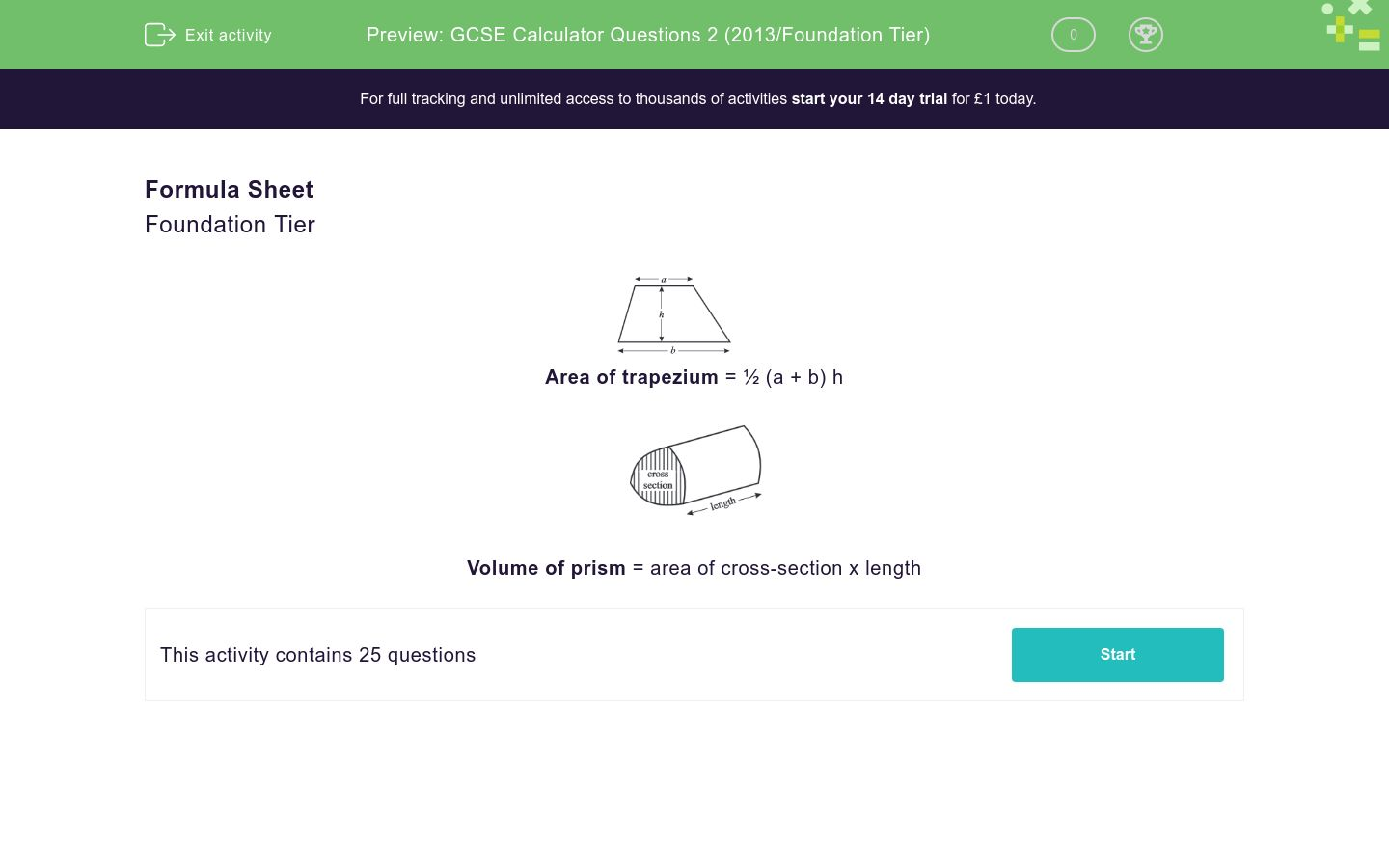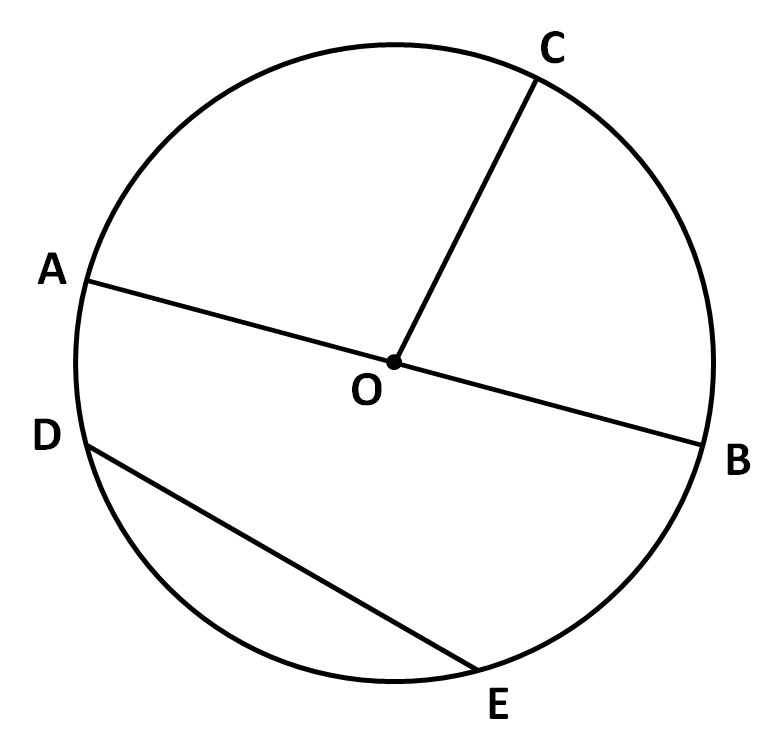# GCSE Calculator Questions 2 (2013/Foundation Tier)

This worksheet contains a sample of practice questions based on the 2013 GCSE Foundation Level syllabus. Calculators may be used.Key stage:  KS 4

Curriculum topic:  GCSE Practice Papers

Curriculum subtopic:  Selection of Topics for Calculator Practice

Difficulty level:### QUESTION 1 of 10

Formula Sheet

Foundation TierArea of trapezium = ½ (a + b) hVolume of prism = area of cross-section x length

Place these numbers against an appropriate description.

8      9      10      36      37      40

## Column B

a square number
8
a cube number
37
a prime number
9
a factor of 20
36
a multiple of 8
40
a multiple of 6
10

The heights in centimetres of ten seedlings were measured as follows.

Work out the range of these heights.

(Just write the number)

1.28,  1.47,  1.89,  1.8,  2.09,  2.5,  1.7,  1.18,  0.16,   2.21

Find the value of the following when a = -3

4a2 - 3a

Kate goes shopping with two £20 notes.

She buys a coffee for £3.20 and lunch for £8.55.

Then she buys a jacket in the sale.

Jacket Sale

Normal Price £28

10% off all prices in the SALE

How much money does she have left?

The perimeter of a square is 62 cm.

Calculate its area in cm2 to 3 sig. figs.

(Just write the number)

Match the following measurements.

## Column B

45 cm
45 cm
45 mm
4.5 m
450 cm
0.45 cm
0.45 m
4.5 cm
0.045 m
4.5 cm
4.5 mm
450 mm

Increase 140 by 25%.

What is the mathematical name of this shape:The formula for the cost of buying a car on credit is:

Cost of buying car = 36 x Monthly payment + Deposit

Find the monthly payment when the cost of buying the car is £12000 and the deposit is £499.80.

Jack drove 250 miles, measured correct to the nearest 10 miles.

What is the least distance Jack could have driven?

(Just write the number)

Work out:

 42 + 43 44

Look at this diagram, which is to have two lines of reflective symmetry.

Which squares should be yellow?A

B

C

D

E

F

G

H

I

J

K

L

M

Look at this diagram, which shows a rectangle ABCD, which is to be rotated through 90º anticlockwise about the point D to give the rectangle A'B'C'D'.

Write down the coordinates of B'.Work out the size of the missing angle shown below.

(Just write the number)(Diagram not drawn accurately)

Solve:

5x + 8 = 45

(Just write the value of x)

A, B, C, D and E are five points on a circle centred at O.Match the letters to the parts of the circle.

## Column B

AB
OC
diameter
DE
arc
Curved part DE
chord

The diagram shows an irregular pentagon.

Work out the perimeter of the rectangle in cm when x = 6.

(Just write the number)A piece of wood is 240 cm long.

It is cut into three pieces in the ratio 3 : 4 : 5.

Work out the length of the shortest piece in cm.

(Just write the number)

Work out the following to 3 decimal places:

 √596 6 + 1.982

A circle of diameter 14 cm is shown in the diagram.Work out the area of the circle in cm2 to 3 sig. figs.

The pie chart shown below shows the results of a survey about favourite crisp flavours.

168 children voted.Work out how many children voted for Cheese and Onion flavoured crisps.

Use Pythagoras' Theorem to calculate the value of x in this right-angled triangle.(This is not drawn accurately)

Look at this timetable.

At what time does the 1510 London Euston train get to Coventry?

(Just write four digits)Simplify:

y2 + 2y2 + 3y2

5y2

6y2

5y6

6y6

3y6

The diagram shows the code of a padlock.The code is a letter followed by a single digit number (e.g. B3).

What is the probability that I will not be able to open the padlock when I select a code at random?

• Question 1

Place these numbers against an appropriate description.

8      9      10      36      37      40

## Column B

a square number
9
a cube number
8
a prime number
37
a factor of 20
10
a multiple of 8
40
a multiple of 6
36
EDDIE SAYS
36 is the only multiple of 6 in the list, so the square number is 9.
• Question 2

The heights in centimetres of ten seedlings were measured as follows.

Work out the range of these heights.

(Just write the number)

1.28,  1.47,  1.89,  1.8,  2.09,  2.5,  1.7,  1.18,  0.16,   2.21

2.34
EDDIE SAYS
Tallest - shortest = 2.5 - 0.16 = 2.34
• Question 3

Find the value of the following when a = -3

4a2 - 3a

45
EDDIE SAYS
4 × (-3) × (-3) - 3 × (-3) = 36 - -9 = 36 + 9 = 45
• Question 4

Kate goes shopping with two £20 notes.

She buys a coffee for £3.20 and lunch for £8.55.

Then she buys a jacket in the sale.

Jacket Sale

Normal Price £28

10% off all prices in the SALE

How much money does she have left?

£ 3.05
£3.05
EDDIE SAYS
She starts with £40.
After coffee and lunch, she has £40 - 3.20 - 8.55 = £28.25
Jacket costs £28 - 2.80 = £25.20
She has £28.25 - 25.20 = £3.05 left.
• Question 5

The perimeter of a square is 62 cm.

Calculate its area in cm2 to 3 sig. figs.

(Just write the number)

240
EDDIE SAYS
Side length = 62 ÷ 4 = 15.5 cm
Area = 15.5 × 15.5 = 240.25 cm²
• Question 6

Match the following measurements.

## Column B

45 cm
450 mm
45 mm
4.5 cm
450 cm
4.5 m
0.45 m
45 cm
0.045 m
4.5 cm
4.5 mm
0.45 cm
EDDIE SAYS
100 cm = 1 m
10 mm = 1 cm
• Question 7

Increase 140 by 25%.

175
EDDIE SAYS
¼ of 140 = 35
140 + 35 = 175
• Question 8

What is the mathematical name of this shape:cone
• Question 9

The formula for the cost of buying a car on credit is:

Cost of buying car = 36 x Monthly payment + Deposit

Find the monthly payment when the cost of buying the car is £12000 and the deposit is £499.80.

£ 319.45
£319.45
EDDIE SAYS
On the calculator, we type 12000 - 499.80 = ÷ 36 =
• Question 10

Jack drove 250 miles, measured correct to the nearest 10 miles.

What is the least distance Jack could have driven?

(Just write the number)

245
EDDIE SAYS
Anything less than 245 would have been rounded to less than 250, e.g. 244 rounds down to 240.
• Question 11

Work out:

 42 + 43 44

0.3125
EDDIE SAYS
42 + 43 = 16 + 64 = 80
80 ÷ 4 to the power 4 = 0.3125
• Question 12

Look at this diagram, which is to have two lines of reflective symmetry.

Which squares should be yellow?A
C
K
EDDIE SAYS
Reflect the yellow square in both lines of symmetry.
• Question 13

Look at this diagram, which shows a rectangle ABCD, which is to be rotated through 90º anticlockwise about the point D to give the rectangle A'B'C'D'.

Write down the coordinates of B'.(10,8)
(10, 8)
EDDIE SAYS
A will be at (10, 10) and B will be 2 units below A.
• Question 14

Work out the size of the missing angle shown below.

(Just write the number)(Diagram not drawn accurately)

137
EDDIE SAYS
360° - 90° - 104° - 29° = 137°
• Question 15

Solve:

5x + 8 = 45

(Just write the value of x)

7.4
EDDIE SAYS
Subtract 8 from both sides to get:
5x = 37
Divide both sides by 5 to get:
x = 7.4
• Question 16

A, B, C, D and E are five points on a circle centred at O.Match the letters to the parts of the circle.

## Column B

AB
diameter
OC
DE
chord
Curved part DE
arc
• Question 17

The diagram shows an irregular pentagon.

Work out the perimeter of the rectangle in cm when x = 6.

(Just write the number)144
EDDIE SAYS
Perimeter = 7x + 2x + 5x + 4x + 6x = 24x
When x = 6, 24x = 144 cm
• Question 18

A piece of wood is 240 cm long.

It is cut into three pieces in the ratio 3 : 4 : 5.

Work out the length of the shortest piece in cm.

(Just write the number)

60
EDDIE SAYS
3 + 4 + 5 = 12 shares
12 shares ≡ 240cm, so 1 share ≡ 240 ÷ 12 = 20 cm
3 shares ≡ 3 × 20 = 60 cm
• Question 19

Work out the following to 3 decimal places:

 √596 6 + 1.982

2.461
EDDIE SAYS
On the calculator, type:
√596 ÷ (6 + 1.98 squared) = 2.460899886...
• Question 20

A circle of diameter 14 cm is shown in the diagram.Work out the area of the circle in cm2 to 3 sig. figs.

154
EDDIE SAYS
Radius is half the diameter = 7 cm
Area = πr² = 49 × π = 153.9cm²
• Question 21

The pie chart shown below shows the results of a survey about favourite crisp flavours.

168 children voted.Work out how many children voted for Cheese and Onion flavoured crisps.

70
EDDIE SAYS
360° ≡ 168 children
1° ≡ 168 ÷ 360 children
150° ≡ 168 ÷ 360 × 150 children
• Question 22

Use Pythagoras' Theorem to calculate the value of x in this right-angled triangle.(This is not drawn accurately)

4.9
EDDIE SAYS
x² + 5² = 7²
x² + 25 = 49
x² = 49 - 25 = 24
x = √24 = 4.898979...
• Question 23

Look at this timetable.

At what time does the 1510 London Euston train get to Coventry?

(Just write four digits)1614
EDDIE SAYS
Use the second column of times.
• Question 24

Simplify:

y2 + 2y2 + 3y2

6y2
EDDIE SAYS
one y² and two more y² and three more y² makes 6y² in total
i.e. collect like terms.
• Question 25

The diagram shows the code of a padlock.The code is a letter followed by a single digit number (e.g. B3).

What is the probability that I will not be able to open the padlock when I select a code at random?

7/8
EDDIE SAYS
There are 8 combinations:
A1, A2, A3, A4
B1, B2, B3, B4
Only one of these will be correct, and so 7 out of 8 are incorrect.
---- OR ----

Sign up for a £1 trial so you can track and measure your child's progress on this activity.

### What is EdPlace?

We're your National Curriculum aligned online education content provider helping each child succeed in English, maths and science from year 1 to GCSE. With an EdPlace account you’ll be able to track and measure progress, helping each child achieve their best. We build confidence and attainment by personalising each child’s learning at a level that suits them.